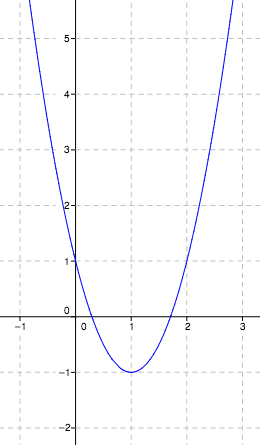### Home > CCA2 > Chapter 12 > Lesson 12.1.3 > Problem12-57

12-57.

Rewrite $f(x) = 2x^2 - 4x + 1$ in graphing form. State its domain, range, vertex and line of symmetry. Include a labeled sketch as part of your solution.

Multiply the equation by 1/2 so that the $x^²$ term has a coefficient of $1$.

$\frac{1}{2}f(x)\ =\ x^2-2x+\frac{1}{2}$

Complete the square to factor the polynomial.

$\frac{1}{2}f(x)\ =\ (x^2-2x+1) +\frac{1}{2}-1$

$\frac{1}{2}f(x)\ =\ (x^2-2x+1) -\frac{1}{2}$

$f(x)\ =\ 2(x-1)^2-1$

Use the graph of the function below to help you find the domain, range, vertex, and line of symmetry.